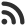Feed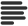Articles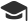Tutorials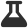CodingLab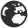CommunityLeaderboardFounder & Programmer at HackersFriend April 20, 2020, 5:55 p.m. ⋅ 335 views

# Advanced python concepts the easy way ( Lambda , Map and Filter )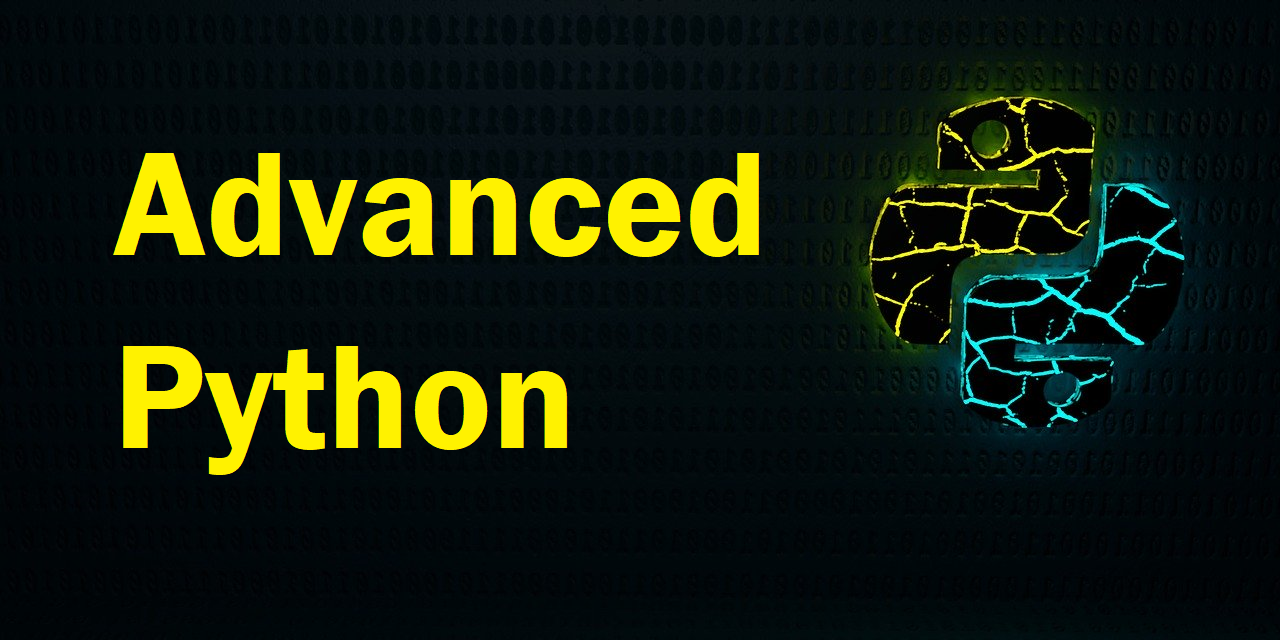This is continuation to previous article on Using advanced Python concepts the easy way.

• Lambda
• Map
• and Filter

## Lambda

Lambda is simply a one-liner function. It can take some arguments and can have one experssion in it to execute. This comes very handy with map and other functions, where we need to execute a one liner experssions in for every element of list.

Syntax begins with a lambda keyword and arguments then expression.

``lambda arguments : expression``

Here is a simple lambda to calculate square of any number.

``````>>> Get_Sqr = lambda x: x * x
>>> Get_Sqr(9)
81

``````

This is equivalent to a function declared normal way, like this:

``````def GetSquare(x):
return x*x

GetSquare(9)``````
``81``

## Map

Map is used to iterate over a list / sequence and apply lambda / function to every element. You can do the same with list comprehensions also.

``map(function or lambda , sequence or list)``

Let's use our previous lambda to print square of every number of a list.

Map will return an iteratanle object, so we need to wrap it a with they type we need. Here we will wrap it with list.

``````arr = [1, 2, 3, 4, 5]
arr = list(map(lambda x : x*x, arr))
print (arr)``````
``[1, 4, 9, 16, 25]``

## Filter

Filter will apply a lambda / function to all the elements of the given sequence and will return an iteratable object of all the elements for which lambda / function returned true.

``filter(function or lambda, sequence or list)``

Let's use filters to get all the odd numbers of a list.

``````arr = [1, 2, 3, 4, 5, 6]
arr = list(filter(lambda x : x%2 != 0, arr))
print (arr)``````
``[1, 3, 5]``

In upcoming article I'll talk about Iteration protocol and generators.

Header image credits:  Image by Brian Ego from Pixabay

 arrow_upward UpvoteComment arrow_downward downvote

Events

Nov. 28, 2018, 5:30 p.m.

Python from zero to hero

place Delhi

Aug. 13, 2018, 5:30 p.m.

Python from zero to hero

place Bangalore ( HackersFriend office BTM Layout)